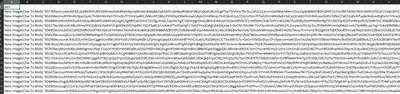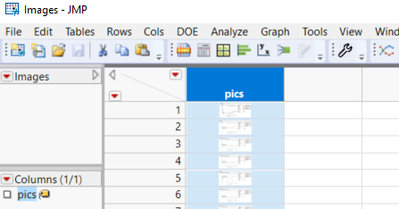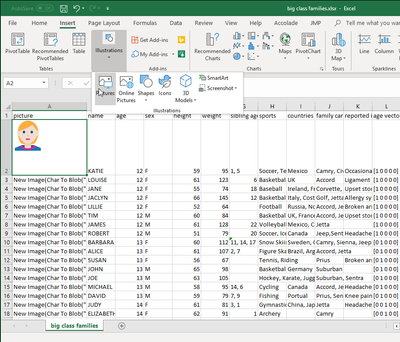Choose Language Hide Translation Bar
Highlighted

## Copying PNG images from JMP file to Excel

I am trying to copy the data from JMP file into Excel.

Here is my script:

When I run the script the data get copied into the Excel but when I open the excel file it shows some garbage value

Can someone help me with this?``````dt = Open( "C:\Jay\JMP\JMP data\Images.jmp" );
OutputFolder = "C:\Jay\JMP\JMP data\Test.xlsx";

ColNames = dt << Get Column Names("String");

DesColumnsList = {"pics"};
for(i = 1, i <= N Cols(dt), i++,
If(Contains(DesColumnsList,ColNames[i]),
Col = Column(dt,i); // Get Reference for Column
Col << Set Selected(1);
);
);

dt_New = dt << Subset(Selected Rows(0),Selected Columns(1));

Close(dt_New,Save(OutputFolder || "Test.xlsx"));``````

Highlighted

## Re: Copying PNG images from JMP file to Excel

Hi @Jacksmith12,

This is a really good question, and I did some searching, but couldn't find a scripting way to evaluate the blob (which is what is written into the excel cell), into an image as it's saving the data table as an excel file. Instead, you can save each individual image in the output directory and then insert them with Excel individually. I tried that with the Big Class Families.jmp file and got this:You might be able to write a Powershell file or some other non-JMP code that loops through the number of rows in the excel file to insert pictures at the appropriate cell.

Here's the code I was playing with to at least get you started -- based on your original section of code:

``````dt = Open( "\$Sample_Data/big class families.jmp" );
OutputFolder = "\Desktop\test"; //or wherever you are saving things

ColNames = dt << Get Column Names("String");

DesColumnsList = {"pics"};
for(i = 1, i <= N Cols(dt), i++,
If(Contains(DesColumnsList,ColNames[i]),
Col = Column(dt,i); // Get Reference for Column
Col << Set Selected(1);
);
);

dt_New = dt << Subset(Selected Rows(0),Selected Columns(1));

Close(dt_New,Save(OutputFolder || "\Test.xlsx"));

For (k=1, k<=N Rows(dt), k++,
dt:picture[k]<<Save Image(OutputFolder || "\image"||char(k)||".png");
);``````

It's really the last For loop that does anything new for you.

I don't know how to automate getting the images INTO excel.

Hopefully this is a start!,

DS

Article Labels

There are no labels assigned to this post.